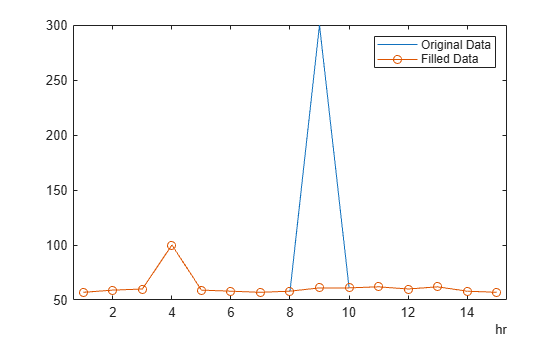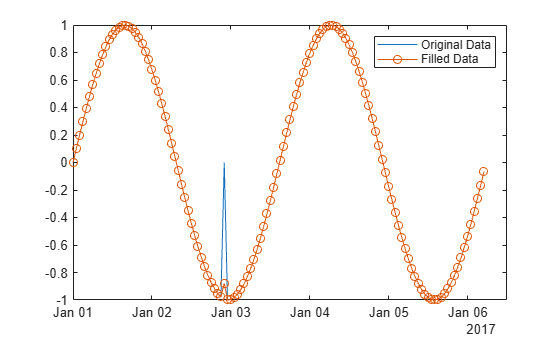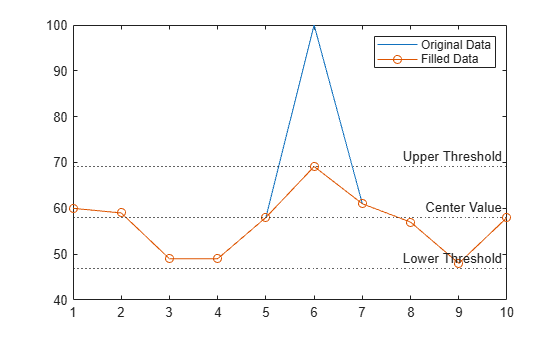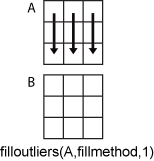# filloutliers

Detect and replace outliers in data

## Syntax

``B = filloutliers(A,fillmethod)``
``B = filloutliers(A,fillmethod,findmethod)``
``B = filloutliers(A,fillmethod,"percentiles",threshold)``
``B = filloutliers(A,fillmethod,movmethod,window)``
``B = filloutliers(___,dim)``
``B = filloutliers(___,Name,Value)``
``[B,TF]= filloutliers(___)``
``````[B,TF,L,U,C] = filloutliers(___)``````

## Description

example

````B = filloutliers(A,fillmethod)` finds outliers in `A` and replaces them according to `fillmethod`. For example, `filloutliers(A,"previous")` replaces outliers with the previous nonoutlier element. If `A` is a matrix, then `filloutliers` operates on each column of `A` separately.If `A` is a multidimensional array, then `filloutliers` operates along the first dimension of `A` whose size does not equal 1. If `A` is a table or timetable, then `filloutliers` operates on each variable of `A` separately. By default, an outlier is a value that is more than three scaled median absolute deviations (MAD) from the median. ```

example

````B = filloutliers(A,fillmethod,findmethod)` specifies a method for detecting outliers. For example, `filloutliers(A,"previous","mean")` defines an outlier as an element of `A` more than three standard deviations from the mean.```
````B = filloutliers(A,fillmethod,"percentiles",threshold)` defines outliers as points outside of the percentiles specified in `threshold`. The `threshold` argument is a two-element row vector containing the lower and upper percentile thresholds, such as `[10 90]`.```

example

````B = filloutliers(A,fillmethod,movmethod,window)` detects local outliers using a moving window mean or median with window length `window`. For example, `filloutliers(A,"previous","movmean",5)` identifies outliers as elements more than three local standard deviations from the local mean within a five-element window.```

example

````B = filloutliers(___,dim)` specifies the dimension of `A` to operate along for any of the previous syntaxes. For example, `filloutliers(A,"linear",2)` operates on each row of a matrix `A`.```

example

````B = filloutliers(___,Name,Value)` specifies additional parameters for detecting and replacing outliers using one or more name-value arguments. For example, `filloutliers(A,"previous","SamplePoints",t)` detects outliers in `A` relative to the corresponding elements of a time vector `t`.```

example

````[B,TF]= filloutliers(___)` also returns a logical array `TF` that indicates the position of the filled elements of `B` that were previously outliers.```

example

``````[B,TF,L,U,C] = filloutliers(___)``` also returns the lower threshold `L`, upper threshold `U`, and center value `C` used by the outlier detection method.```

## Examples

collapse all

Fill outliers in a vector of data using the `"linear"` method, and visualize the filled data.

Create a vector of data containing two outliers.

`A = [57 59 60 100 59 58 57 58 300 61 62 60 62 58 57];`

Replace the outliers using linear interpolation.

`B = filloutliers(A,"linear");`

Plot the original data and the data with the outliers filled.

```plot(A) hold on plot(B,"o-") legend("Original Data","Filled Data")```Identify potential outliers in a table of data, fill any outliers using the `"nearest"` fill method, and visualize the cleaned data.

Create a timetable of data, and visualize the data to detect potential outliers.

```T = hours(1:15); V = [57 59 60 100 59 58 57 58 300 61 62 60 62 58 57]; A = timetable(T',V'); plot(A.Time,A.Var1)```

Fill outliers in the data, where an outlier is defined as a point more than three standard deviations from the mean. Replace the outlier with the nearest element that is not an outlier.

`B = filloutliers(A,"nearest","mean")`
```B=15×1 timetable Time Var1 _____ ____ 1 hr 57 2 hr 59 3 hr 60 4 hr 100 5 hr 59 6 hr 58 7 hr 57 8 hr 58 9 hr 61 10 hr 61 11 hr 62 12 hr 60 13 hr 62 14 hr 58 15 hr 57 ```

In the same graph, plot the original data and the data with the outlier filled.

```hold on plot(B.Time,B.Var1,"o-") legend("Original Data","Filled Data")```Use a moving median to detect and fill local outliers within a sine wave that corresponds to a time vector.

Create a vector of data containing a local outlier.

```x = -2*pi:0.1:2*pi; A = sin(x); A(47) = 0;```

Create a time vector that corresponds to the data in `A`.

`t = datetime(2017,1,1,0,0,0) + hours(0:length(x)-1);`

Define outliers as points more than three local scaled MAD from the local median within a sliding window. Find the location of the outlier in `A` relative to the points in `t` with a window size of 5 hours. Fill the outlier with the computed threshold value using the method `"clip"`.

`[B,TF,L,U,C] = filloutliers(A,"clip","movmedian",hours(5),"SamplePoints",t);`

Plot the original data and the data with the outlier filled.

```plot(t,A) hold on plot(t,B,"o-") legend("Original Data","Filled Data")```Create a matrix of data containing outliers along the diagonal.

`A = randn(5,5) + diag(1000*ones(1,5))`
```A = 5×5 103 × 1.0005 -0.0013 -0.0013 -0.0002 0.0007 0.0018 0.9996 0.0030 -0.0001 -0.0012 -0.0023 0.0003 1.0007 0.0015 0.0007 0.0009 0.0036 -0.0001 1.0014 0.0016 0.0003 0.0028 0.0007 0.0014 1.0005 ```

Fill outliers with zeros based on the data in each row, and display the new values.

```[B,TF] = filloutliers(A,0,2); B```
```B = 5×5 0 -1.3077 -1.3499 -0.2050 0.6715 1.8339 0 3.0349 -0.1241 -1.2075 -2.2588 0.3426 0 1.4897 0.7172 0.8622 3.5784 -0.0631 0 1.6302 0.3188 2.7694 0.7147 1.4172 0 ```

You can access the detected outlier values and their filled values using `TF` as an index vector.

`[A(TF) B(TF)]`
```ans = 5×2 103 × 1.0005 0 0.9996 0 1.0007 0 1.0014 0 1.0005 0 ```

Create a vector containing two outliers and detect their locations.

```A = [57 59 60 100 59 58 57 58 300 61 62 60 62 58 57]; detect = isoutlier(A)```
```detect = 1x15 logical array 0 0 0 1 0 0 0 0 1 0 0 0 0 0 0 ```

Fill the outliers using the `"nearest"` method. Instead of using a detection method, provide the outlier locations detected by `isoutlier`.

`B = filloutliers(A,"nearest","OutlierLocations",detect)`
```B = 1×15 57 59 60 59 59 58 57 58 61 61 62 60 62 58 57 ```

Replace the outlier in a vector of data using the `"clip"` fill method.

Create a vector of data with an outlier.

`A = [60 59 49 49 58 100 61 57 48 58];`

Detect outliers with the default method `"median"`, and replace the outlier with the upper threshold value by using the `"clip"` fill method.

`[B,TF,L,U,C] = filloutliers(A,"clip");`

Plot the original data, the data with the outlier filled, and the thresholds and center value determined by the outlier detection method. The center value is the median of the data, and the upper and lower thresholds are three scaled MAD above and below the median.

```plot(A) hold on plot(B,"o-") yline([L U C],":",["Lower Threshold","Upper Threshold","Center Value"]) legend("Original Data","Filled Data")```## Input Arguments

collapse all

Input data, specified as a vector, matrix, multidimensional array, table, or timetable.

• If `A` is a table, then its variables must be of type `double` or `single`, or you can use the `DataVariables` argument to list `double` or `single` variables explicitly. Specifying variables is useful when you are working with a table that contains variables with data types other than `double` or `single`.

• If `A` is a timetable, then `filloutliers` operates only on the table elements. If row times are used as sample points, then they must be unique and listed in ascending order.

Data Types: `double` | `single` | `table` | `timetable`

Fill method for replacing outliers, specified as one of these values.

Fill MethodDescription
Numeric scalarSpecified scalar value
`"center"`Center value determined by `findmethod`
`"clip"`Lower threshold value for elements smaller than the lower threshold determined by `findmethod`; upper threshold value for elements larger than the upper threshold determined by `findmethod`
`"previous"`Previous nonoutlier value
`"next"`Next nonoutlier value
`"nearest"`Nearest nonoutlier value
`"linear"`Linear interpolation of neighboring, nonoutlier values
`"spline"`Piecewise cubic spline interpolation
`"pchip"`Shape-preserving piecewise cubic spline interpolation
`"makima"`Modified Akima cubic Hermite interpolation (numeric, `duration`, and `datetime` data types only)

Data Types: `double` | `single` | `char` | `string`

Method for detecting outliers, specified as one of these values.

MethodDescription
`"median"`Outliers are defined as elements more than three scaled MAD from the median. The scaled MAD is defined as `c*median(abs(A-median(A)))`, where `c=-1/(sqrt(2)*erfcinv(3/2))`.
`"mean"`Outliers are defined as elements more than three standard deviations from the mean. This method is faster but less robust than `"median"`.
`"quartiles"`Outliers are defined as elements more than 1.5 interquartile ranges above the upper quartile (75 percent) or below the lower quartile (25 percent). This method is useful when the data in `A` is not normally distributed.
`"grubbs"`Outliers are detected using Grubbs’ test, which removes one outlier per iteration based on hypothesis testing. This method assumes that the data in `A` is normally distributed.
`"gesd"`Outliers are detected using the generalized extreme Studentized deviate test for outliers. This iterative method is similar to `"grubbs"` but can perform better when multiple outliers are masking each other.

Percentile thresholds, specified as a two-element row vector whose elements are in the interval [0,100]. The first element indicates the lower percentile threshold, and the second element indicates the upper percentile threshold. The first element of `threshold` must be less than the second element.

For example, a threshold of `[10 90]` defines outliers as points below the 10th percentile and above the 90th percentile.

Moving method for detecting outliers, specified as one of these values.

MethodDescription
`"movmedian"`Outliers are defined as elements more than three local scaled MAD from the local median over a window length specified by `window`. This method is also known as a Hampel filter.
`"movmean"`Outliers are defined as elements more than three local standard deviations from the local mean over a window length specified by `window`.

Window length, specified as a positive integer scalar, a two-element vector of positive integers, a positive duration scalar, or a two-element vector of positive durations.

When `window` is a positive integer scalar, the window is centered about the current element and contains `window-1` neighboring elements. If `window` is even, then the window is centered about the current and previous elements.

When `window` is a two-element vector of positive integers `[b f]`, the window contains the current element, `b` elements backward, and `f` elements forward.

When `A` is a timetable or `SamplePoints` is specified as a `datetime` or `duration` vector, `window` must be of type `duration`, and the windows are computed relative to the sample points.

Operating dimension, specified as a positive integer scalar. If no value is specified, then the default is the first array dimension whose size does not equal 1.

Consider an `m`-by-`n` input matrix, `A`:

• `filloutliers(A,fillmethod,1)` fills outliers according to the data in each column of `A` and returns an `m`-by-`n` matrix.• `filloutliers(A,fillmethod,2)` fills outliers according to the data in each row of `A` and returns an `m`-by-`n` matrix.For table or timetable input data, `dim` is not supported and operation is along each table or timetable variable separately.

### Name-Value Arguments

Specify optional pairs of arguments as `Name1=Value1,...,NameN=ValueN`, where `Name` is the argument name and `Value` is the corresponding value. Name-value arguments must appear after other arguments, but the order of the pairs does not matter.

Example: `filloutliers(A,"center","mean",ThresholdFactor=4)`

Before R2021a, use commas to separate each name and value, and enclose `Name` in quotes.

Example: `filloutliers(A,"center","mean","ThresholdFactor",4)`

Data Options

collapse all

Sample points, specified as a vector of sample point values or one of the options in the following table when the input data is a table. The sample points represent the x-axis locations of the data, and must be sorted and contain unique elements. Sample points do not need to be uniformly sampled. The vector ```[1 2 3 ...]``` is the default.

When the input data is a table, you can specify the sample points as a table variable using one of these options.

Indexing SchemeExamples

Variable name:

• A string scalar or character vector

• `"A"` or `'A'` — A variable named `A`

Variable index:

• An index number that refers to the location of a variable in the table

• A logical vector. Typically, this vector is the same length as the number of variables, but you can omit trailing `0` or `false` values

• `3` — The third variable from the table

• `[false false true]` — The third variable

Function handle:

• A function handle that takes a table variable as input and returns a logical scalar

• `@isnumeric` — One variable containing numeric values

Variable type:

• `vartype("numeric")` — One variable containing numeric values

Note

This name-value argument is not supported when the input data is a `timetable`. Timetables use the vector of row times as the sample points. To use different sample points, you must edit the timetable so that the row times contain the desired sample points.

Moving windows are defined relative to the sample points. For example, if `t` is a vector of times corresponding to the input data, then `filloutliers(rand(1,10),"previous","movmean",3,"SamplePoints",t)` has a window that represents the time interval between `t(i)-1.5` and `t(i)+1.5`.

When the sample points vector has data type `datetime` or `duration`, the moving window length must have type `duration`.

Example: ```filloutliers([1 100 3 4],"nearest","SamplePoints",[1 2.5 3 4])```

Example: `filloutliers(T,"nearest","SamplePoints","Var1")`

Data Types: `single` | `double` | `datetime` | `duration`

Table variables to operate on, specified as one of the options in this table. The `DataVariables` value indicates which variables of the input table to fill. The data type associated with the indicated variables must be `double` or `single`.

Other variables in the table not specified by `DataVariables` pass through to the output without being filled.

Indexing SchemeExamples

Variable names:

• A string, character vector, or cell array

• A `pattern` object

• `"A"` or `'A'` — A variable named `A`

• `["A","B"]` or `{'A','B'}` — Two variables named `A` and `B`

• `"Var"+digitsPattern(1)` — Variables named `"Var"` followed by a single digit

Variable index:

• An index number that refers to the location of a variable in the table

• A vector of numbers

• A logical vector. Typically, this vector is the same length as the number of variables, but you can omit trailing `0` or `false` values

• `3` — The third variable from the table

• `[2 3]` — The second and third variables from the table

• `[false false true]` — The third variable

Function handle:

• A function handle that takes a table variable as input and returns a logical scalar

• `@isnumeric` — All the variables containing numeric values

Variable type:

• `vartype("numeric")` — All the variables containing numeric values

Example: ```filloutliers(A,"previous","DataVariables",["Var1" "Var2" "Var4"])```

Replace values indicator, specified as one of these logical or numeric values when `A` is a table or timetable:

• `true` or `1` — Replace input table variables containing outliers with filled table variables.

• `false` or `0` — Append the input table with all table variables that were checked for outliers. The outliers in the appended variables are filled.

For vector, matrix, or multidimensional array input data, `ReplaceValues` is not supported.

Example: `filloutliers(T,"previous","ReplaceValues",false)`

Outlier Detection Options

collapse all

Detection threshold factor, specified as a nonnegative scalar.

For methods `"median"` and `"movmedian"`, the detection threshold factor replaces the number of scaled MAD, which is 3 by default.

For methods `"mean"` and `"movmean"`, the detection threshold factor replaces the number of standard deviations from the mean, which is 3 by default.

For methods `"grubbs"` and `"gesd"`, the detection threshold factor is a scalar ranging from 0 to 1. Values close to 0 result in a smaller number of outliers, and values close to 1 result in a larger number of outliers. The default detection threshold factor is 0.05.

For the `"quartiles"` method, the detection threshold factor replaces the number of interquartile ranges, which is 1.5 by default.

This name-value argument is not supported when the specified method is `"percentiles"`.

Maximum filled outliers by GESD, specified as a positive integer scalar. The `MaxNumOutliers` value specifies the maximum number of outliers that are filled by the `"gesd"` method. For example, `filloutliers(A,"linear","gesd","MaxNumOutliers",5)` fills no more than five outliers.

The default value for `MaxNumOutliers` is the integer nearest to 10 percent of the number of elements in `A`. Setting a larger value for the maximum number of outliers makes it more likely that all outliers are detected but at the cost of reduced computational efficiency.

The `"gesd"` method assumes the nonoutlier input data is sampled from an approximate normal distribution. When the data is not sampled in this way, the number of filled outliers might exceed the `MaxNumOutliers` value.

Known outlier indicator, specified as a logical vector, matrix, or multidimensional array of the same size as `A`. The known outlier indicator elements can be `true` to indicate an outlier in the corresponding location of `A` or `false` otherwise. When you specify `OutlierLocations`, `filloutliers` does not use an outlier detection method. Instead, it uses the elements of the known outlier indicator to define outliers. The output `TF` contains the same logical vector, matrix, or multidimensional array.

You cannot specify the `OutlierLocations` name-value argument if you specify `findmethod`.

Data Types: `logical`

## Output Arguments

collapse all

Filled data, returned as a vector, matrix, multidimensional array, table, or timetable.

`B` is the same size as `A` unless the value of `ReplaceValues` is `false`. If the value of `ReplaceValues` is `false`, then the width of `B` is the sum of the input data width and the number of data variables specified.

Filled data indicator, returned as a vector, matrix, or multidimensional array. Elements with a value of 1 (`true`) correspond to filled elements of `B` that were previously outliers. Elements with a value of 0 (`false`) correspond to unchanged elements.

`TF` is the same size as `B`.

Data Types: `logical`

Lower threshold used by the outlier detection method, returned as a scalar, vector, matrix, multidimensional array, table, or timetable. For example, the lower threshold value of the default outlier detection method is three scaled MAD below the median of the input data.

If `findmethod` is used for outlier detection, then `L` has the same size as `A` in all dimensions except for the operating dimension where the length is 1. If `movmethod` is used, then `L` has the same size as `A`.

Upper threshold used by the outlier detection method, returned as a scalar, vector, matrix, multidimensional array, table, or timetable. For example, the upper threshold value of the default outlier detection method is three scaled MAD above the median of the input data.

If `findmethod` is used for outlier detection, then `U` has the same size as `A` in all dimensions except for the operating dimension where the length is 1. If `movmethod` is used, then `U` has the same size as `A`.

Center value used by the outlier detection method, returned as a scalar, vector, matrix, multidimensional array, table, or timetable. For example, the center value of the default outlier detection method is the median of the input data.

If `findmethod` is used for outlier detection, then `C` has the same size as `A` in all dimensions except for the operating dimension where the length is 1. If `movmethod` is used, then `C` has the same size as `A`.

The scaled MAD is defined as `c*median(abs(A-median(A)))`, where `c=-1/(sqrt(2)*erfcinv(3/2))`.